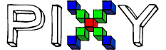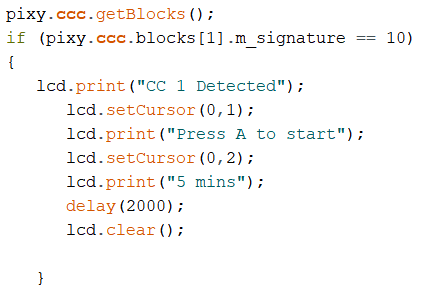# Converting Octal to Decimal for CC signature does not work

Hello I’m trying to use the the CC signatureThe normal signature works but the CC signature doesn’t in my example i have m_signature == 10 which came from CC = 12 which is octal and converted it to decimal = 10. is there a way or a specific code for reading CC signatures? because i want to detect a CC signature then print some text on my LCD, is there a way for me to do this? Thank you in advance!

Hello,
This tends to confuse people quite a bit. Just remember that numbers are numbers and that 12 octal is exactly the same as 10 decimal. When programming the Arduino, you can actually put a 0 in front of a number and the C compiler will treat it as octal. So 012==10 is a true statement. (That is, 12(octal) is equal to 10(decimal)) You can also bring up a converter https://www.rapidtables.com/convert/number/decimal-to-octal.html

if you prefer that things be represented in decimal.

Hope this helps.

Edward